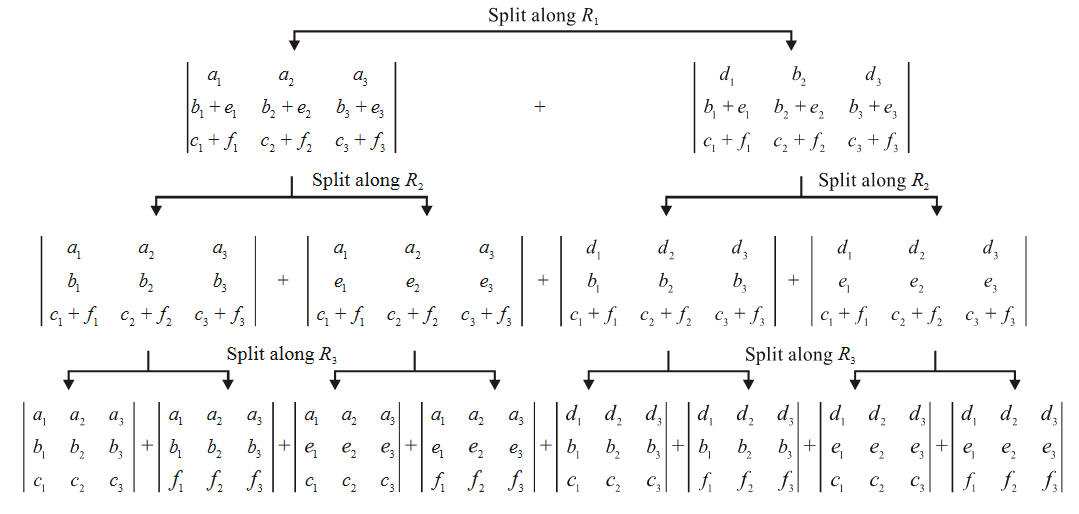# Basic Properties of Determinants

## Basic Properties of Determinants, JEE Syllabus

Over the next few pages, we are going to see that to evaluate a determinant, it is not always necessary to fully expand it. There are a number of properties of determinants, particularly row and column transformations, that can simplify the evaluation of any determinant considerably. We are going to discuss these properties one by one and also work out as many examples as we can. The discussion will generally involve 3 × 3 determinants. The first three properties have already been mentioned in the first exercise.

Property 1 : The value of determinant is not changed when rows are changed into columns and columns into rows.

For example,

$\Delta =\left| \ \begin{matrix} {{a}_{1}} & {{a}_{2}} & {{a}_{3}} \\ {{b}_{1}} & {{b}_{2}} & {{b}_{3}} \\ {{c}_{1}} & {{c}_{2}} & {{c}_{3}} \\\end{matrix}\ \right|\ \ =\ \ \left| \ \begin{matrix} {{a}_{1}} & {{b}_{1}} & {{c}_{1}} \\ {{a}_{2}} & {{b}_{2}} & {{c}_{2}} \\ {{a}_{3}} & {{b}_{3}} & {{c}_{3}} \\\end{matrix}\ \right|$

A possible justification can be obtained by expanding the first determinant along R1 and the second along C1; the resulting expansions are the same. Verify this

Property 2 : If any two rows or columns of a determinant are interchanged, the sign of the determinant changes but its magnitude remains the same:

For example,

$\Delta =\left| \ \begin{matrix} {{a}_{1}} & {{a}_{2}} & {{a}_{3}} \\ {{b}_{1}} & {{b}_{2}} & {{b}_{3}} \\ {{c}_{1}} & {{c}_{2}} & {{c}_{3}} \\\end{matrix}\ \right|\ \ =\ \ -\ \left| \ \begin{matrix} {{a}_{1}} & {{a}_{2}} & {{a}_{3}} \\ {{c}_{1}} & {{c}_{2}} & {{c}_{3}} \\ {{b}_{1}} & {{b}_{2}} & {{b}_{3}} \\\end{matrix}\ \right|$

This should be obvious: Expanding the first determinant along R1, we have

\begin{align} \Delta &={{a}_{1}}\left| \begin{matrix} {{b}_{2}} & {{b}_{3}} \\ {{c}_{2}} & {{c}_{3}} \\\end{matrix} \right|\ -{{a}_{2}}\left| \begin{matrix} {{b}_{1}} & {{b}_{3}} \\ {{c}_{1}} & {{c}_{3}} \\\end{matrix} \right|+{{a}_{3}}\left| \begin{matrix} {{b}_{1}} & {{b}_{2}} \\ {{c}_{1}} & {{c}_{2}} \\ \end{matrix} \right| \\\\ & =-\left[ {{a}_{1}}\left| \begin{matrix} {{c}_{2}} & {{c}_{3}} \\ {{b}_{2}} & {{b}_{3}} \\\end{matrix} \right|\ -{{a}_{2}}\left| \begin{matrix} {{c}_{1}} & {{c}_{3}} \\ {{b}_{1}} & {{b}_{3}} \\\end{matrix} \right|+{{a}_{3}}\left| \begin{matrix} {{c}_{1}} & {{c}_{2}} \\ {{b}_{1}} & {{b}_{2}} \\\end{matrix} \right| \right] \\\\ & =-\left| \begin{matrix} {{a}_{1}} & {{a}_{2}} & {{a}_{3}} \\ {{c}_{1}} & {{c}_{2}} & {{c}_{3}} \\ {{b}_{1}} & {{b}_{2}} & {{b}_{3}} \\\end{matrix} \right| \\ \end{align}

Similarly, it can be shown that a column interchange leads to a – sign.

Property - 3 : A determinant having two rows or two columns identical has the value zero

For example,

\begin{align} \Delta& =\left| \ \begin{matrix} p & q & r \\ p & q & r \\ x & y & z \\\end{matrix}\ \right|\ =p\left| \ \begin{matrix} q & r \\ y & z \\\end{matrix}\ \right|-q\left| \ \begin{matrix} p & r \\ x & z \\\end{matrix}\ \right|+\left| \ \begin{matrix} q & q \\ x & y \\\end{matrix}\ \right| \\ \\ & =0 \\ \end{align}

Alternatively, if we exchange the 1st and 2nd rows, $$\Delta$$ stays the same, but by the previous property, it should be $$-\Delta$$ , so

\begin{align} \Delta &=-\Delta \\ \Rightarrow \quad \Delta &=0 \\ \end{align}

Property - 4 : Multiplying all the elements of a row (or column) by a scalar (a real number) is equivalent to multiplying the determinant by that scalar.

For example,

$\Delta =\left| \ \begin{matrix} \lambda {{a}_{1}} & \lambda {{a}_{2}} & \lambda {{a}_{3}} \\ {{b}_{1}} & {{b}_{2}} & {{b}_{3}} \\ {{c}_{1}} & {{c}_{2}} & {{c}_{3}} \\\end{matrix}\ \right|\ \ =\lambda \ \left| \ \begin{matrix} {{a}_{1}} & {{a}_{2}} & {{a}_{3}} \\{{b}_{1}} & {{b}_{2}} & {{b}_{3}} \\ {{c}_{1}} & {{c}_{2}} & {{c}_{3}} \\\end{matrix}\ \right|$

Note carefully that  $$\lambda$$ is multiplied with elements of just one row and not of the entire determinant. Another example:

$\left| \ \begin{matrix} \lambda {{a}_{1}} & \lambda {{a}_{2}} & \lambda {{a}_{3}} \\ \lambda {{b}_{1}} & \lambda {{b}_{2}} & \lambda {{b}_{3}} \\ \lambda {{c}_{1}} & \lambda {{c}_{2}} & \lambda {{c}_{3}} \\\end{matrix}\ \right|\ \ =\ \ {{\lambda }^{3}}\ \left| \ \begin{matrix} {{a}_{1}} & {{a}_{2}} & {{a}_{3}} \\ {{b}_{1}} & {{b}_{2}} & {{b}_{3}} \\ {{c}_{1}} & {{c}_{2}} & {{c}_{3}} \\\end{matrix}\ \right|$

This property is trivial and can be proved easily by expansion

Property - 5 : A determinant can be split into a sum of two determinants along any row or column

For example,

$\left| \ \begin{matrix} {{a}_{1}}+{{d}_{1}} & {{a}_{2}}+{{d}_{2}} & {{a}_{3}}+{{d}_{3}} \\ {{b}_{1}} & {{b}_{2}} & {{b}_{3}} \\ {{c}_{1}} & {{c}_{2}} & {{c}_{3}} \\\end{matrix}\ \right|\ \ =\ \ \ \left| \ \begin{matrix} {{a}_{1}} & {{a}_{2}} & {{a}_{3}} \\ {{b}_{1}} & {{b}_{2}} & {{b}_{3}} \\ {{c}_{1}} & {{c}_{2}} & {{c}_{3}} \\\end{matrix}\ \right|\ +\ \left| \ \begin{matrix} {{d}_{1}} & {{d}_{2}} & {{d}_{3}} \\ {{b}_{1}} & {{b}_{2}} & {{b}_{3}} \\ {{c}_{1}} & {{c}_{2}} & {{c}_{3}} \\\end{matrix}\ \right|\ \$

The property is evident by expanding the determinant on the LHS along R1.

Let us do an example using this property.

Example - 3

Simplify

$\Delta =\left| \ \begin{matrix} {{a}_{1}}+{{d}_{1}} & {{a}_{2}}+{{d}_{2}} & {{a}_{3}}+{{d}_{3}} \\ {{b}_{1}}+{{c}_{1}} & {{b}_{2}}+{{c}_{2}} & {{b}_{3}}+{{c}_{3}} \\ {{c}_{1}}+{{f}_{1}} & {{c}_{2}}+{{f}_{2}} & {{c}_{3}}+{{f}_{3}} \\\end{matrix}\ \right|$

by splitting it into simpler determinants

Solution: If you think that the answer is

$\Delta =\left| \ \begin{matrix} {{a}_{1}} & {{a}_{2}} & {{a}_{3}} \\ {{b}_{1}} & {{b}_{2}} & {{b}_{3}} \\ {{c}_{1}} & {{c}_{2}} & {{c}_{3}} \\\end{matrix}\ \right|\ +\ \left| \ \begin{matrix} {{d}_{1}} & {{d}_{2}} & {{d}_{3}} \\ {{e}_{1}} & {{e}_{2}} & {{e}_{3}} \\ {{f}_{1}} & {{f}_{2}} & {{f}_{3}} \\\end{matrix}\ \right|\$

then you are mistaken, for splitting a determinant into a sum of two determinants can be done along only one row or one column at a time.

So here’s what we’ll do : split $$\Delta$$ along R1, then split the resulting two determinants along R2 to obtain four determinants, and finally split these four determinants along R3 to obtain eight determinants: